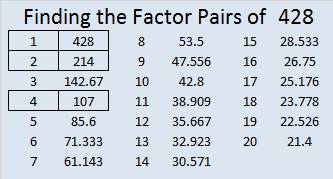# 428 St. Patrick’s Day Smile

Happy Saint Patrick’s Day! Today’s puzzle reminds me of a smile, and I hope it brightens your day.

428 is divisible by 4 because 28 is divisible by 4.

Since 28 can be evenly divided by 4, but not by 8, we know that ANY number ending in 128, 328, 528, 728, and 928 will also be divisible by 8. Notice all those odd digits just before the 28? Likewise, we know that ANY number ending in 028, 228, 428, 628, or 828 will NOT be divisible by 8.

All of the factors of 428 are listed below today’s  puzzle.Print the puzzles or type the factors on this excel file:10 Factors 2015-03-16

• 428 is a composite number.
• Prime factorization: 428 = 2 x 2 x 107, which can be written 428 = (2^2) x 107
• The exponents in the prime factorization are 2 and 1. Adding one to each and multiplying we get (2 + 1)(1 + 1) = 3 x 2  = 6. Therefore 428 has exactly 6 factors.
• Factors of 428: 1, 2, 4, 107, 214, 428
• Factor pairs: 428 = 1 x 428, 2 x 214, or 4 x 107
• Taking the factor pair with the largest square number factor, we get √428 = (√4)(√107) = 2√107 ≈ 20.6882This site uses Akismet to reduce spam. Learn how your comment data is processed.